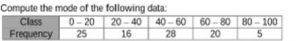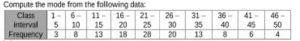RS Aggarwal Class 10 Solutions Chapter 9 - Mean Median Mode Ex 9C(9.3)

RS Aggarwal Class 10 Chapter 9 - Mean Median Mode Ex 9C(9.3) Solutions Free PDF

RS Aggarwal Solutions for Class 10 can prove to be an extremely important study resource for students looking to improve their performance in the exam. It helps students to clear all their doubts and provide a deeper understanding of the subject. With these solutions, they learn to solve difficult questions easily and enhance their problem-solving skills. Thus, these solutions act as a potent exam preparation material to score excellent marks in the exam.

For Class 10 students, it is important to refer to RS Aggarwal Class 10 Solution to strengthen your concepts and revise important topics thoroughly. All the exercise questions solutions provided here are simpler and can be practiced easily to clear the concepts.

Download PDF of RS Aggarwal Class 10 Solutions Chapter 9– Mean Median Mode Ex 9C (9.3)

Question-1: Find the mode of the following frequency distribution:

 Marks 10-20 20-30 30-40 40-50 50-60 Frequency 12 35 45 25 13

As the class 50-60 has maximum frequency, so it is the modal class:

Therefore, $x_{k}=50,h=10,f_{k}=20\:and\:f_{k-1}=13\:and\:f_{k+1}=6$

$Mode\:M_{o}=x_{k}+\left [ h\times \frac{\left ( f_{k}-f_{k-1} \right )}{\left ( 2f_{k}-f_{k-1} -f_{k+1}\right )} \right ]$

$=50+\left [ 10\times \frac{\left ( 20-13 \right )}{\left ( 2\times 20-13 -6\right )} \right ]$

= 50 +(70/21) = (50+3.33) = 53.33

Hence, mode = 53.33

Question-2:As the class 40 – 60 has maximum frequency, so it is the modal class:

Therefore, $x_{k}=40,h=10,f_{k}=62\:and\:f_{k-1}=47\:and\:f_{k+1}=37$

$Mode\:M_{o}=x_{k}+\left [ h\times \frac{\left ( f_{k}-f_{k-1} \right )}{\left ( 2f_{k}-f_{k-1} -f_{k+1}\right )} \right ]$

$=40+\left [ 10\times \frac{\left ( 62-47 \right )}{\left ( 2\times 62-47 -37\right )} \right ]$

= 40 + (150/40) = (40+3.75) = 43.75

Hence, mode = 43.75

Question-3: Find the mode of the following distribution:

 Class interval 10-14 14-18 18-22 22-26 26-30 30-34 24-38 38-42 Frequency 8 6 11 20 25 22 10 4

As the class 26 – 30 has maximum frequency, so it is the modal class:

Therefore, $x_{k}=26,h=4,f_{k}=25\:and\:f_{k-1}=20\:and\:f_{k+1}=22$

$Mode\:M_{o}=x_{k}+\left [ h\times \frac{\left ( f_{k}-f_{k-1} \right )}{\left ( 2f_{k}-f_{k-1} -f_{k+1}\right )} \right ]$

$=26+\left [4\times \frac{\left ( 25-20 \right )}{\left ( 2\times 25-20-22\right )} \right ]$

= 26 + (5/2) = (26+2.5) = 28.5

ence, mode = 28.5

Question-4: Given below is the distribution of total household expenditure of 200 manual workers in a city.

 Expenditure (in rs.) No. of manual workers 1000-1500 24 1500-2000 40 2000-2500 31 2500-3000 28 3000-3500 32 3500-4000 23 4000-4500 17 4500-5000 5

Find the expenditure done by a maximum number of manual workers.

As the class 1500 – 2000 has maximum frequency, so it is the modal class:

Therefore, $x_{k}=1500,h=500,f_{k}=40\:and\:f_{k-1}=24\:and\:f_{k+1}=31$

$Mode\:M_{o}=x_{k}+\left [ h\times \frac{\left ( f_{k}-f_{k-1} \right )}{\left ( 2f_{k}-f_{k-1} -f_{k+1}\right )} \right ]$

$=1500+\left [500\times \frac{\left (40-24 \right )}{\left ( 2\times 40-24-31\right )} \right ]$

= 1500 + (500 x 16/25)

= (1500+320) = Rs 1820

Hence, the average expenditure done by maximum number of workers = Rs. 1820

Question-5: Calculate the mode from the following data:

 Monthly salary (in rs.) No. of employees 0-5000 90 5000-10000 150 10000-15000 100 15000-20000 80 20000-25000 70 25000-30000 10

As the class 5000 – 10000 has maximum frequency, so it is the modal class:

Therefore, $x_{k}=5000,h=5000,f_{k}=150\:and\:f_{k-1}=90\:and\:f_{k+1}=100$

$Mode\:M_{o}=x_{k}+\left [ h\times \frac{\left ( f_{k}-f_{k-1} \right )}{\left ( 2f_{k}-f_{k-1} -f_{k+1}\right )} \right ]$

$=5000+\left [5000\times \frac{\left (150-90 \right )}{\left ( 2\times 300-90-100\right )} \right ]$

= 5000 + 2727.27

= Rs.7727.27

Hence, mode = Rs.7727.27

Question-6: Compute the mode from the following data:

 Age (in years) 0-5 5-10 10-15 15-20 20-25 25-30 30-35 Number of patients 6 11 18 24 17 13 5

As the class 15-20 has maximum frequency, so it is the modal class:

Therefore, $x_{k}=15,h=5,f_{k}=24\:and\:f_{k-1}=18\:and\:f_{k+1}=17$

$Mode\:M_{o}=x_{k}+\left [ h\times \frac{\left ( f_{k}-f_{k-1} \right )}{\left ( 2f_{k}-f_{k-1} -f_{k+1}\right )} \right ]$

$=15+\left [5\times \frac{\left (24-18 \right )}{\left ( 2\times 48-18-17\right )} \right ]$

= (15+2.30) = 17.3 years

Hence, mode = 17.3 years

Question-7: Compute the mode from the following series:

 Size 45-55 55-65 65-75 75-85 85-95 95-105 105-115 Frequency 7 12 17 30 32 6 10

As the class 85-95 has maximum frequency, so it is the modal class:

Therefore, $x_{k}=85,h=10,f_{k}=32\:and\:f_{k-1}=30\:and\:f_{k+1}=6$

$Mode\:M_{o}=x_{k}+\left [ h\times \frac{\left ( f_{k}-f_{k-1} \right )}{\left ( 2f_{k}-f_{k-1} -f_{k+1}\right )} \right ]$

$=85+\left [10\times \frac{\left ( 32-30 \right )}{\left ( 2\times 64-30-6\right )} \right ]$

= 85 + (5/7) = (85+0.71) = 85.71

Hence, mode = 85.71

Question-8:The given series is converted from inclusive to exclusive form and on preparing the frequency table, we get

 Class Frequency 0.5-5.5 3 5.5-10.5 8 10.5-15.5 13 15.5-20.5 18 20.5-25.5 28 25.5-30.5 20 30.5-35.5 13 35.5-40.5 8 40.5-45.5 6 45.5-50.5 3

As the class 20.5-25.5 has maximum frequency, so it is the modal class:

Therefore, $x_{k}=20.5,h=5,f_{k}=28\:and\:f_{k-1}=18\:and\:f_{k+1}=20$

$Mode\:M_{o}=x_{k}+\left [ h\times \frac{\left ( f_{k}-f_{k-1} \right )}{\left ( 2f_{k}-f_{k-1} -f_{k+1}\right )} \right ]$

$=20.5+\left [5\times \frac{\left (28-18 \right )}{\left ( 2\times 56-18-20\right )} \right ]$

= 20.5 + [5 x 10 /18]

= 20.5 + 2.78 = 23.28

Hence, mode = 23.28

EXERCISE – 9D

Question-1: Find the mean, mode and median of the following frequency distribution:

 Class 0-10 10-20 20-30 30-40 40-50 50-60 60-70 Frequency 4 4 7 10 12 8 5

Let assumed mean be 35, h = 10, now we have

 Class $Frequency\:f_{i}$ $Mid value\:x_{i}$ $u_{i}=\left ( \frac{x_{i}-A}{h} \right )$ C.F $f_{i}u_{i}$ 0-10 5 5 -3 5 -15 10-20 10 15 -2 15 -20 20-30 18 25 -1 33 -18 30-40 30 35-A 0 63 0 40-50 20 45 1 83 20 50-60 12 55 2 95 24 60-70 5 65 3 100 15 N= 100 $\sum \left ( f_{i}u_{i} \right )=6$

(i) Mean

= $\bar{x}=A+h\left ( \frac{\sum f_{i}u_{i}}{N} \right )$

= 35 + 10 x (6/100) = 35 + 0.6 = 35.6

(ii) N = 100, N/2 = 50

Cumulative frequency just after 50 is 63

Median class is 30-40

I = 30, h = 10, N = 100, c = 33, f = 30

Therefore, $Median\:M_{e}=I+h\left ( \frac{\frac{N}{2}-c}{f} \right )=30+10\left ( \frac{50-33}{30} \right )$

= 30 + 10 (17/30) = 30 + 5.67 = 35.67

(iii) Mode = 3 x median – 2 x mean

= 3 x 35.67 – 2 x 35.6 = 107.01 – 71.2

= 35.81

Thus, Mean = 35.6, Median = 35.67 and Mode = 35.81

Question-2:

Let us assume mean A be 8.5. Class interval h = 3

 Class $Frequency\:f_{i}$ $Mid value\:x_{i}$ $u_{i}=\left ( \frac{x_{i}-A}{h} \right )$ $f_{i}u_{i}$ C.F 1-4 6 2.5 -2 -12 6 4-7 30 5.5 -1 -30 36 7-10 40 8.5 = A 0 0 76 10-13 16 11.5 1 16 92 13-16 4 14.5 2 8 96 16-19 4 17.5 3 12 100 N=100 $\sum \left ( f_{i}u_{i} \right )=6$

N = total frequency = 100

(i) Mean

= $\bar{x}=A+h\left ( \frac{\sum f_{i}u_{i}}{N} \right )$

= 8.5+3 x -6/100 = 8.5-18/100

= 8.5-0.18 = 8.32

(ii) N/2 = 50, Cumulative frequency just after 50 is 76

Median class is 7-10

I=7, h=3, N=100, f =40,c=36

Therefore, $Median\:M_{e}=I+h\left ( \frac{\frac{N}{2}-c}{f} \right )=7+3\left ( \frac{50-36}{40} \right )$

= 7+3 x 14/40 = 7+21/20 = 7+1.05 = 8.05

(iii) Mode = 3 x median – 2 x mean

= 3 x 8.05 – 2 x 8.32 = 24.15 – 16.64

= 7.51

Thus, mean = 8.32, median = 8.05, mode = 7.51

Question-3: A survey regarding the heights (in cm) of 50 girls of a class was conducted and the following data was obtained:

 Class 120-130 130-140 140-150 150-160 160-170 Total Frequency 2 8 12 20 8 30

Find the mean, median and mode of the above data.

Let the assumed mean A be 145. Class interval h = 10.

 Class $Frequency\:f_{i}$ $Mid value\:x_{i}$ $u_{i}=\left ( \frac{x_{i}-A}{h} \right )$ $f_{i}u_{i}$ C.F 120-130 2 125 -2 -4 2 130-140 8 135 -1 -4 10 140-150 12 145 = A 0 0 22 150-160 20 155 1 20 42 160-170 8 165 2 16 50 N=50 $\sum \left ( f_{i}u_{i} \right )=24$

(i) Mean

= $\bar{x}=A+h\left ( \frac{\sum f_{i}u_{i}}{N} \right )$

= 145+10 x (24/50)

=145+4.8 = 149.8

(ii) N = 50, N/2 = 25

Cumulative frequency just after 25 is 42

Median class is 150-160

I = 150, h = 10, N = 50, c = 22, f = 20

Therefore, $Median\:M_{e}=I+h\left ( \frac{\frac{N}{2}-c}{f} \right )=150+10\left ( \frac{25-22}{20} \right )$

= 30 + 10 x 3/20 = 150 + 1.5 = 151.5

(iii) Mode = 3 x median – 2 x mean

= 3 x 151.5 – 2 x 149.8 = 454.5 – 299.6

= 154.9

Thus, mean = 149.8, median = 151.5, mode = 154.9

Question-4: The following table gives the daily income of 50 workers of a factory:

 Daily income (in rs.) 100-120 120-140 140-160 160-180 180-200 Number of workers 12 14 8 6 10

Find the mean, median and mode of the above data.

Let assumed mean A = 150 and h = 20

 Class $Frequency\:f_{i}$ $Mid value\:x_{i}$ $u_{i}=\left ( \frac{x_{i}-A}{h} \right )$ $f_{i}u_{i}$ C.F 100-120 12 110 -2 -24 12 120-140 14 130 -1 -14 26 140-160 8 150 = A 0 0 34 160-180 6 170 1 6 40 180-200 10 190 2 20 50 N = 50 $\sum \left ( f_{i}u_{i} \right )=-12$

(i) Mean

= $\bar{x}=A+h\left ( \frac{\sum f_{i}u_{i}}{N} \right )$

= 150-24/5 = 150-4.8 = 145.2

(ii) N = 50, N/2 = 25

Cumulative frequency just after 25 is 26

Median class is 120-140

I = 120, h = 10, N = 50, c = 12, f = 14

Therefore, $Median\:M_{e}=I+h\left ( \frac{\frac{N}{2}-c}{f} \right )=120+20\left ( \frac{25-12}{14} \right )$

= 120+20 x 13/14 = 120+130/7 = 120+18.6 = 138.6

(iii) Mode = 3 x median – 2 x mean

= 3 x 138.6 – 2 x 145.2

= 415.8 – 190.4 = 125.4

Thus, mean = 145.2, median = 138.6, mode = 125.4

Question-5: The table below shows the daily expenditure on food of 30 households in a locality:

 Daily expenditure (in rs.) Number of households 100-150 6 150-200 7 200-250 12 250-300 3 300-350 2

Find the mean, median and mode of the above data/p>

Let assumed mean A = 225 and h = 50

 Class $Frequency\:f_{i}$ $Mid value\:x_{i}$ $u_{i}=\left ( \frac{x_{i}-A}{h} \right )$ $f_{i}u_{i}$ C.F 100-150 6 125 2 12 6 150-200 7 175 1 7 13 200-250 12 225 0 0 25 250-300 3 275 1 3 28 300-350 2 325 2 4 30 N = 30 $\sum \left ( f_{i}u_{i} \right )=-12$

(i) Mean

= $\bar{x}=A+h\left ( \frac{\sum f_{i}u_{i}}{N} \right )$

= 225 – 20 = 205

(ii) N = 30, N/2 = 15

Cumulative frequency just after 15 is 25

Corresponding frequency interval is 200 – 250

Median class is 200 – 250

Cumulative frequency c just before this class = 13

So I = 200, f = 12, N/2 = 15, h = 50, c = 13

Therefore, $Median\:M_{e}=I+h\left ( \frac{\frac{N}{2}-c}{f} \right )=200+50\left ( \frac{15-13}{12} \right )$

= 200+50 x 2/12 = 200+25/3 = 200+8.33 = 208.33

Hence, Mean = 205 and Median = 208.33

Key Features of RS Aggarwal Class 10 Solutions Chapter 9 –  Mean Median Mode Ex 9C (9.3)

•  It is prepared as per the latest syllabus of the Class 10 maths.
• It improves your problem-solving skills and you can evaluate your weaknesses and strength.
• Solving the RS Aggarwal maths solution will help you to cover the entire syllabus.
• It is considered as the best reference material while preparing for the exam.

Practise This Question

For how long time period should a flow of 1019 electrons be maintained between two points A and B to have a constant current of 8 A between them?